Share

# RD Sharma solutions for Class 8 Mathematics chapter 20 - Mensuration - I (Area of a Trapezium and a Polygon)

## Mathematics for Class 8 by R D Sharma (2019-2020 Session)

#### RD Sharma Mathematics Class 8 by R D Sharma (2019-2020 Session)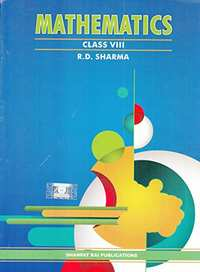## Chapter 20: Mensuration - I (Area of a Trapezium and a Polygon)

Ex. 20.10Ex. 20.20Ex. 20.30

#### Chapter 20: Mensuration - I (Area of a Trapezium and a Polygon) Exercise 20.10 solutions [Pages 13 - 15]

Ex. 20.10 | Q 1 | Page 13

A flooring tile has the shape of a parallelogram whose base is 24 cm and the corresponding height is 10 cm. How many such tiles are required to cover a floor of area 1080 m2?

Ex. 20.10 | Q 2 | Page 13

A plot is in the form of a rectangle ABCD having semi-circle on BC as shown in Fig. 20.23. If AB = 60 m and BC = 28 m, find the area of the plot.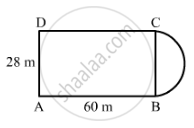Ex. 20.10 | Q 3 | Page 13

A playground has the shape of a rectangle, with two semi-circles on its smaller sides as diameters, added to its outside. If the sides of the rectangle are 36 m and 24.5 m, find the area of the playground. (Take π = 22/7).

Ex. 20.10 | Q 4 | Page 13

A rectangular piece is 20 m long and 15 m wide. From its four corners, quadrants of radii 3.5 m have been cut. Find the area of the remaining part.

Ex. 20.10 | Q 5 | Page 14

The inside perimeter of a running track (shown in Fig. 20.24) is 400 m. The length of each of the straight portion is 90 m and the ends are semi-circles. If track is everywhere 14 m wide, find the area of the track. Also, find the length of the outer running track.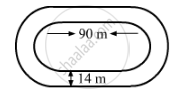Ex. 20.10 | Q 6 | Page 14

Find the area of Fig. 20.25, in square cm, correct to one place of decimal. (Take π = 22/7)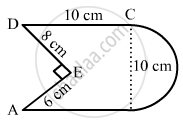Ex. 20.10 | Q 7 | Page 14

The diameter of a wheel of a bus is 90 cm which makes 315 revolutions per minute. Determine its speed in kilometres per hour. [Use π = 22/7]

Ex. 20.10 | Q 8 | Page 14

The area of a rhombus is 240 cm2 and one of the diagonal is 16 cm. Find another diagonal.

Ex. 20.10 | Q 9 | Page 14

The diagonals of a rhombus are 7.5 cm and 12 cm. Find its area.

Ex. 20.10 | Q 10 | Page 14

The diagonal of a quadrilateral shaped field is 24 m and the perpendiculars dropped on it from the remaining opposite vertices are 8 m and 13 m. Find the area of the field.

Ex. 20.10 | Q 11 | Page 14

Find the area of a rhombus whose side is 6 cm and whose altitude is 4 cm. If one of its diagonals is 8 cm long, find the length of the other diagonal.

Ex. 20.10 | Q 12 | Page 14

The floor of a building consists of 3000 tiles which are rhombus shaped and each of its diagonals are 45 cm and 30 cm in length. Find the total cost of polishing the floor, if the cost per m2 is Rs 4.

Ex. 20.10 | Q 13 | Page 14

A rectangular grassy plot is 112 m long and 78 m broad. It has a gravel path 2.5 m wide all around it on the side. Find the area of the path and the cost of constructing it at Rs 4.50 per square metre.

Ex. 20.10 | Q 14 | Page 14

Find the area of a rhombus, each side of which measures 20 cm and one of whose diagonals is 24 cm.

Ex. 20.10 | Q 15 | Page 14

The length of a side of a square field is 4 m. what will be the altitude of the rhombus, if the area of the rhombus is equal to the square field and one of its diagonal is 2 m?

Ex. 20.10 | Q 16 | Page 14

Find the area of the field in the form of a rhombus, if the length of each side be 14 cm and the altitude be 16 cm.

Ex. 20.10 | Q 17 | Page 14

The cost of fencing a square field at 60 paise per metre is Rs 1200. Find the cost of reaping the field at the rate of 50 paise per 100 sq. metres.

Ex. 20.10 | Q 18 | Page 14

In exchange of a square plot one of whose sides is 84 m, a man wants to buy a rectangular plot 144 m long and of the same area as of the square plot. Find the width of the rectangular plot.

Ex. 20.10 | Q 19 | Page 14

The area of a rhombus is 84 m2. If its perimeter is 40 m, then find its altitude.

Ex. 20.10 | Q 20 | Page 14

A garden is in the form of a rhombus whose side is 30 metres and the corresponding altitude is 16 m. Find the cost of levelling the garden at the rate of Rs 2 per m2.

Ex. 20.10 | Q 21 | Page 14

A field in the form of a rhombus has each side of length 64 m and altitude 16 m. What is the side of a square field which has the same area as that of a rhombus?

Ex. 20.10 | Q 22 | Page 15

The area of a rhombus is equal to the area of a triangle whose base and the corresponding altitude are 24.8 cm and 16.5 cm respectively. If one of the diagonals of the rhombus is 22 cm, find the length of the other diagonal.

#### Chapter 20: Mensuration - I (Area of a Trapezium and a Polygon) Exercise 20.20 solutions [Pages 22 - 24]

Ex. 20.20 | Q 1.1 | Page 22

Find the area, in square metres, of the trapezium whose bases and altitude is as under:
bases = 12 dm and 20 dm, altitude = 10 dm

Ex. 20.20 | Q 1.2 | Page 22

Find the area, in square metres, of the trapezium whose bases and altitude is as under:
bases = 28 cm and 3 dm, altitude = 25 cm

Ex. 20.20 | Q 1.3 | Page 22

Find the area, in square metres, of the trapezium whose bases and altitude is as under:
bases = 8 m and 60 dm, altitude = 40 dm

Ex. 20.20 | Q 1.4 | Page 22

Find the area, in square metres, of the trapezium whose bases and altitude is as under:
bases = 150 cm and 30 dm, altitude = 9 dm.

Ex. 20.20 | Q 2 | Page 22

Find the area of trapezium with base 15 cm and height 8 cm, if the side parallel to the given base is 9 cm long.

Ex. 20.20 | Q 3 | Page 22

Find the area of a trapezium whose parallel sides are of length 16 dm and 22 dm and whose height is 12 dm.

Ex. 20.20 | Q 4 | Page 22

Find the height of a trapezium, the sum of the lengths of whose bases (parallel sides) is 60 cm and whose area is 600 cm2.

Ex. 20.20 | Q 5 | Page 22

Find the altitude of a trapezium whose area is 65 cm2 and whose bases are 13 cm and 26 cm.

Ex. 20.20 | Q 6 | Page 22

Find the sum of the lengths of the bases of a trapezium whose area is 4.2 m2 and whose height is 280 cm.

Ex. 20.20 | Q 7.1 | Page 22

Find the area of a trapezium whose parallel sides of lengths 10 cm and 15 cm are at a distance of 6 cm from each other. Calculate this area as
the sum of the areas of two triangles and one rectangle.

Ex. 20.20 | Q 7.2 | Page 22

Find the area of a trapezium whose parallel sides of lengths 10 cm and 15 cm are at a distance of 6 cm from each other. Calculate this area as the difference of the area of a rectangle and the sum of the areas of two triangles.

Ex. 20.20 | Q 8 | Page 22

The area of a trapezium is 960 cm2. If the parallel sides are 34 cm and 46 cm, find the distance between them.

Ex. 20.20 | Q 9 | Page 23

Find the area of Fig. 20.35 as the sum of the areas of two trapezium and a rectangle.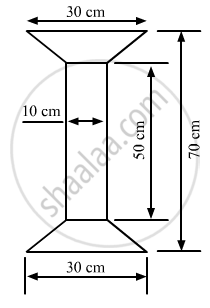Ex. 20.20 | Q 10 | Page 23

Top surface of a table is trapezium in shape. Find its area if its parallel sides are 1 m and 1.2 m and perpendicular distance between them is 0.8 m.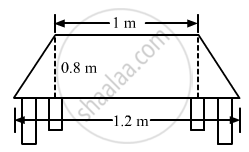Ex. 20.20 | Q 11 | Page 23

The cross-section of a canal is a trapezium in shape. If the canal is 10 m wide at the top 6 m wide at the bottom and the area of cross-section is 72 m2 determine its depth.

Ex. 20.20 | Q 12 | Page 23

The area of a trapezium is 91 cm2 and its height is 7 cm. If one of the parallel sides is longer than the other by 8 cm, find the two parallel sides.

Ex. 20.20 | Q 13 | Page 23

The area of a trapezium is 384 cm2. Its parallel sides are in the ratio 3 : 5 and the perpendicular distance between them is 12 cm. Find the length of each one of the parallel sides.

Ex. 20.20 | Q 14 | Page 23

Mohan wants to buy a trapezium shaped field. Its side along the river is parallel and twice the side along the road. If the area of this field is 10500 m2 and the perpendicular distance between the two parallel sides is 100 m, find the length of the side along the river.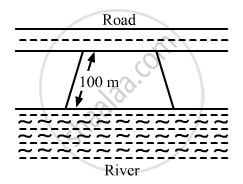Ex. 20.20 | Q 15 | Page 24

The area of a trapezium is 1586 cm2 and the distance between the parallel sides is 26 cm. If one of the parallel sides is 38 cm, find the other.

Ex. 20.20 | Q 16 | Page 24

The parallel sides of a trapezium are 25 cm and 13 cm; its nonparallel sides are equal, each being 10 cm, find the area of the trapezium.

Ex. 20.20 | Q 17 | Page 24

Find the area of a trapezium whose parallel sides are 25 cm, 13 cm and the other sides are 15 cm each.

Ex. 20.20 | Q 18 | Page 24

If the area of a trapezium is 28 cm2 and one of its parallel sides is 6 cm, find the other parallel side if its altitude is 4 cm.

Ex. 20.20 | Q 19 | Page 24

In Fig. 20.38, a parallelogram is drawn in a trapezium, the area of the parallelogram is 80 cm2, find the area of the trapezium.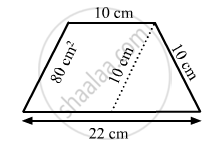Ex. 20.20 | Q 20 | Page 24

Find the area of the field shown in Fig. 20.39 by dividing it into a square, a rectangle and a trapezium.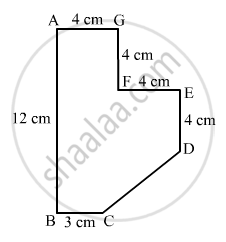#### Chapter 20: Mensuration - I (Area of a Trapezium and a Polygon) Exercise 20.30 solutions [Pages 28 - 29]

Ex. 20.30 | Q 1 | Page 28

Find the area of the pentagon shown in fig. 20.48, if AD = 10 cm, AG = 8 cm, AH = 6 cm, AF = 5 cm, BF = 5 cm, CG = 7 cm and EH = 3 cm.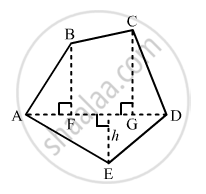Ex. 20.30 | Q 2 | Page 28

Find the area enclosed by each of the following figures [Fig. 20.49 (i)-(iii)] as the sum of the areas of a rectangle and a trapezium: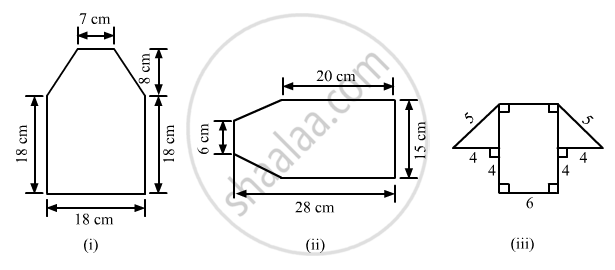Ex. 20.30 | Q 3 | Page 28

There is a pentagonal shaped park as shown in Fig. 20.50. Jyoti and Kavita divided it in two different ways.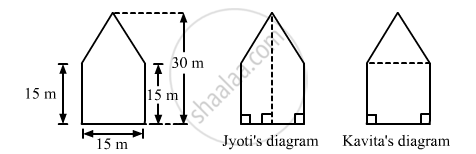Find the area of this park using both ways. Can you suggest some another way of finding its areas?

Ex. 20.30 | Q 4 | Page 29

Find the area of the following polygon, if AL = 10 cm, AM = 20 cm, AN = 50 cm, AO = 60 cm and AD = 90 cm.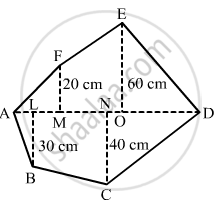Ex. 20.30 | Q 5 | Page 29

Find the area of the following regular hexagon.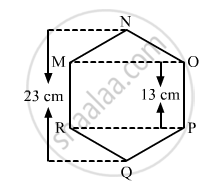## Chapter 20: Mensuration - I (Area of a Trapezium and a Polygon)

Ex. 20.10Ex. 20.20Ex. 20.30

#### RD Sharma Mathematics Class 8 by R D Sharma (2019-2020 Session)## RD Sharma solutions for Class 8 Mathematics chapter 20 - Mensuration - I (Area of a Trapezium and a Polygon)

RD Sharma solutions for Class 8 Maths chapter 20 (Mensuration - I (Area of a Trapezium and a Polygon)) include all questions with solution and detail explanation. This will clear students doubts about any question and improve application skills while preparing for board exams. The detailed, step-by-step solutions will help you understand the concepts better and clear your confusions, if any. Shaalaa.com has the CBSE Mathematics for Class 8 by R D Sharma (2019-2020 Session) solutions in a manner that help students grasp basic concepts better and faster.

Further, we at Shaalaa.com provide such solutions so that students can prepare for written exams. RD Sharma textbook solutions can be a core help for self-study and acts as a perfect self-help guidance for students.

Concepts covered in Class 8 Mathematics chapter 20 Mensuration - I (Area of a Trapezium and a Polygon) are Area of Trapezium, Area of a General Quadrilateral, Area of a Polygon, Concept of Solid Shapes, Concept of Cuboid, Concept of Cube, Concept of Cylinders, Conept of Cylinder, Volume and Capacity, Introduction of Mensuration, Concept of Cuboid, Concept of Cube.

Using RD Sharma Class 8 solutions Mensuration - I (Area of a Trapezium and a Polygon) exercise by students are an easy way to prepare for the exams, as they involve solutions arranged chapter-wise also page wise. The questions involved in RD Sharma Solutions are important questions that can be asked in the final exam. Maximum students of CBSE Class 8 prefer RD Sharma Textbook Solutions to score more in exam.

Get the free view of chapter 20 Mensuration - I (Area of a Trapezium and a Polygon) Class 8 extra questions for Maths and can use Shaalaa.com to keep it handy for your exam preparation

S